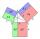# Pythagorean theorem - examples - page 3

1. Widescreen monitorComputer business hit by a wave of widescreen monitors and televisions. Calculate the area of ​​the LCD monitor with a diagonal size 20 inches at ratio 4:3 and then 16:9 aspect ratio. Is buying widescreen monitors with same diagonal more advantageous tha
2. Square diagonalCalculate length of the square diagonal if the perimeter is 476 cm.
3. TriangleTriangle KLM is given by plane coordinates of vertices: K[-12, -11] L[-15, -18] M[-13, -12]. Calculate its area and itsinterior angles.
4. RightDetermine angles of the right triangle with the hypotenuse c and legs a, b, if: ?
5. PrismRight angle prism, whose base is right triangle with leg a = 7 cm and hypotenuse c = 10 cm has same volume as a cube with an edge length of 1 dm. a) Determine the height of the prism b) Calculate the surface of the prism c) What percentage of the cube
6. IS trapezoidCalculate the length of diagonal u and height v of isosceles trapezoid ABCD, whose bases have lengths a = |AB| = 37 cm, c = |CD| = 29 cm and legs b = d = |BC| = |AD| = 28 cm.
7. StepsHow many steps you save if you go square estate for diagonal (crosswise), rather than circumvent on the two sides of its perimeter with 307 steps.
8. ConeCalculate volume and surface area of ​​the cone with diameter of the base d = 15 cm and side of cone with the base has angle 52°.
9. RailwaysRailways climb 7.4 ‰. Calculate the height difference between two points on the railway distant 3539 meters.
10. Right triangleLegs of right are in ratio a:b = 6:8. Hypotenuse has a length of 61 cm. Calculate the perimeter and area of the triangle.
11. Spherical capFrom the sphere of radius 18 was truncated spherical cap. Its height is 12. What part of the volume is spherical cap from whole sphere?
12. RhombusIt is given a rhombus of side length a = 29 cm. Touch points of inscribed circle divided his sides into sections a1 = 14 cm and a2 = 15 cm. Calculate the radius r of the circle and the length of the diagonals of the rhombus.
13. RectangleCalculate the length of the side GN and diagonal QN of rectangle QGNH when given: |HN| = 25 cm and angle ∠ QGH = 28 degrees.
14. Rhombus and inscribed circleIt is given a rhombus with side a = 6 cm and the radius of the inscribed circle r = 2 cm. Calculate the length of its two diagonals.
15. Isosceles right triangleCalculate the area of an isosceles right triangle whose perimeter is 377 cm.
16. R triangleCalculate the area of a right triangle whose longer leg is 6 dm shorter than the hypotenuse and 3 dm longer than the shorter leg.
17. Floating barrelBarrel (cylinder shape) floats on water, top of barrel is 8 dm above water and the width of surfaced barrel part is 23 dm. Barrel length is 24 dm. Calculate the volume of the barrel.
18. LawsFrom which law follows directly the validity of Pythagoras' theorem in the right triangle? ?
19. DiagonalDetermine the dimensions of the cuboid, if diagonal long 53 dm has angle with one edge 42° and with other edge 64°.
20. MediansCalculate the sides of a right triangle if the length of the medians to the legs are ta = 21 cm and tb=12 cm.

Do you have an interesting mathematical example that you can't solve it? Enter it, and we can try to solve it.

To this e-mail address, we will reply solution; solved examples are also published here. Please enter e-mail correctly and check whether you don't have a full mailbox.

Pythagorean theorem is the base for the right triangle calculator.